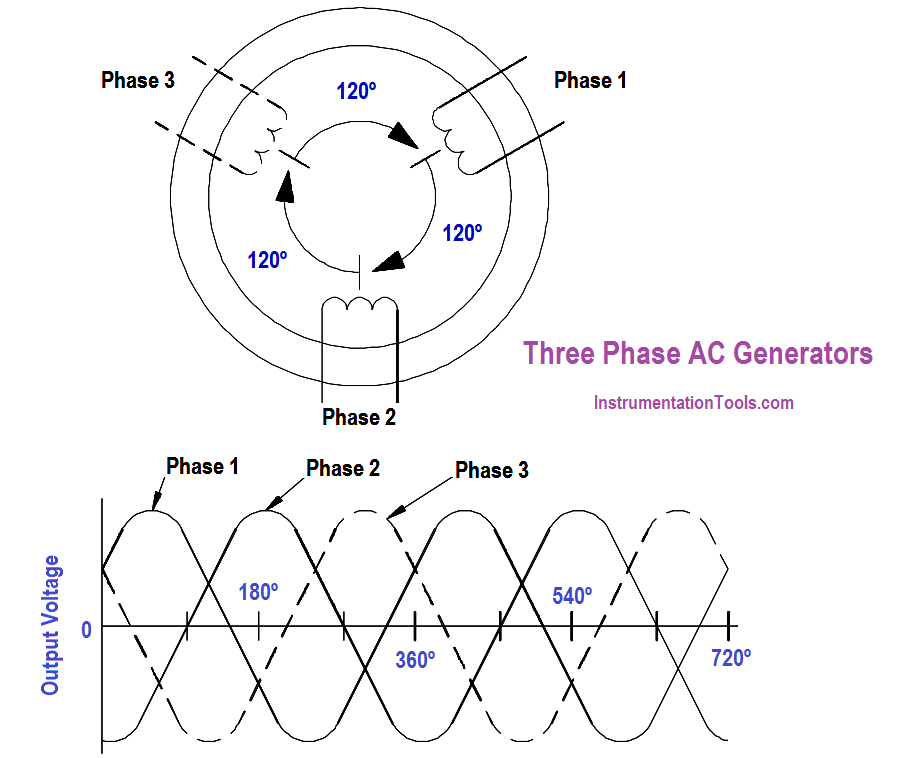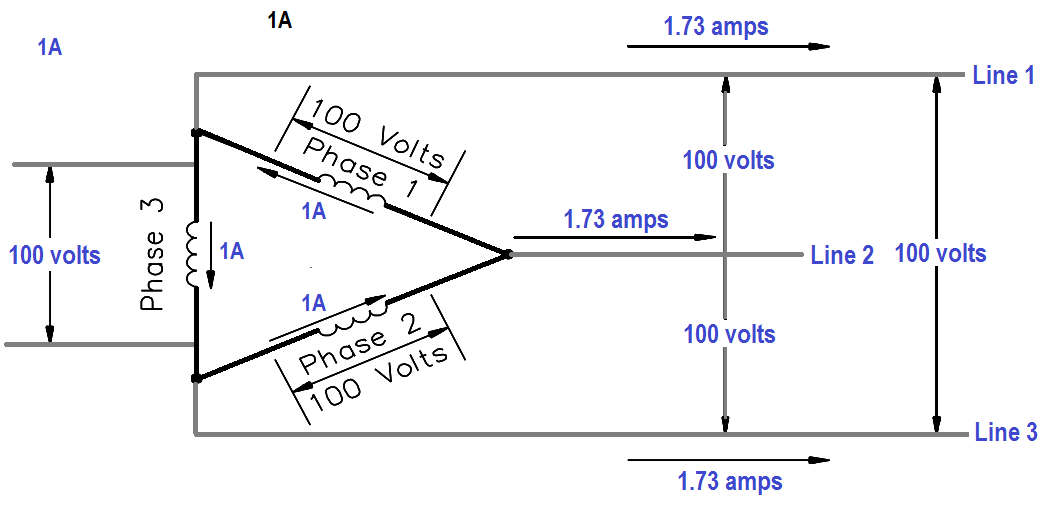# Three Phase AC Generators

The principles of a three-phase generator are basically the same as that of a single-phase generator, except that there are three equally-spaced windings and three output voltages that are all 120° out of phase with one another. Physically adjacent loops (below Figure) are separated by 60° of rotation; however, the loops are connected to the slip rings in such a manner that there are 120 electrical degrees between phases.

The individual coils of each winding are combined and represented as a single coil. The significance of below Figure is that it shows that the three-phase generator has three separate armature windings that are 120 electrical degrees out of phase.Figure : Stationary Armature 3φ Generator

#### AC Generator Connections

As shown in above Figure, there are six leads from the armature of a three-phase generator, and the output is connected to an external load. In actual practice, the windings are connected together, and only three leads are brought out and connected to the external load.

Two means are available to connect the three armature windings. In one type of connection, the windings are connected in series, or delta-connected (∆) (below Figure).Figure : Delta Connection

In a delta-connected generator, the voltage between any two of the phases, called line voltage, is the same as the voltage generated in any one phase.

As shown in Below Figure, the three phase voltages are equal, as are the three line voltages. The current in any line is √3 times the phase current. You can see that a delta-connected generator provides an increase in current, but no increase in voltage.Figure : Characteristics of a Delta-Connected Generator

An advantage of the delta-connected AC generator is that if one phase becomes damaged or open, the remaining two phases can still deliver three-phase power. The capacity of the generator is reduced to 57.7% of what it was with all three phases in operation.

In the other type of connection, one of the leads of each winding is connected, and the remaining three leads are connected to an external load. This is called a wye connection (Y) (Below Figure).Figure : Wye Connection

The voltage and current characteristics of the wye-connected AC generator are opposite to that of the delta connection. Voltage between any two lines in a wye-connected AC generator is 1.73 (or ) 3 times any one phase voltage, while line currents are equal to phase currents. The wye-connected AC generator provides an increase in voltage, but no increase in current ( below Figure).Figure : Characteristics of a Wye-Connected AC Generator

An advantage of a wye-connected AC generator is that each phase only has to carry 57.7% of line voltage and, therefore, can be used for high voltage generation.